# CBSE Solutions for Class 10 English

#### GSEB std 10 science solution for Gujarati check Subject Chapters Wise::

Find the common difference of the AP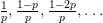. (2013D)

જવાબ :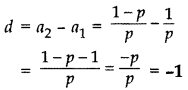Find the common difference of the A.P.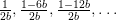. (2013D)

જવાબ : d = a2 – a1 =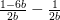=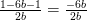= -3

Find the common difference of the A.P.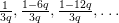. (2013D)

જવાબ : d = a2 – a1 =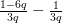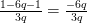= -2

Calculate the common difference of the A.P.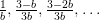. (2013D)

જવાબ : d = a2 – a1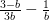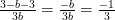Calculate the common difference of the A.P.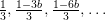(2013OD)

જવાબ : d = a2 – a1 =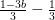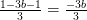= -b

What is the common difference of an A.P. in which a21 – a7 = 84? (2017OD )

જવાબ : a21 – a7 = 84 …[Given in the question
∴ (a + 20d) – (a + 6d) = 84 …[an = a + (n – 1)d
20d – 6d = 84
14d = 84 ⇒ d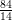= 6

Find the 9th term from the end (towards the first term) of the A.P.    5,9,13, …, 185. (2016D)

જવાબ : Here, a = 5, d = 9 – 5 = 4, l = 185
9th term from the end = 185 – (9 – 1)4
= 185 – 8 × 4 = 185 – 32 = 153

The angles of a triangle are in A.P., the least being half the greatest. Find the angles. (2011D)

જવાબ : 40°, 60°, 80°

Is -150  a term of the A.P. 17, 12, 7, 2, … ? (2011D)

જવાબ : no

Which term of the progression 4, 9, 14, 19, … is 109? (2011D)

જવાબ : 22nd term

Which term of the progression 20, 192, 183, 17 … is the first negative term? (2017OD)

જવાબ : 28th term

The 4th term of an A.P. is zero. Will the 25th term of the A.P. is three times its 11th term. (2016OD)

જવાબ : Yes Let 1st term = a, Common difference = d
a4 = 0 a + 3d = 0 ⇒ a = -3d … (A)
To prove: a25 = 3 × a11
a + 24d = 3(a + 10d) …[From (A)
⇒ -3d + 24d = 3(-3d + 10d)
⇒ 21d = 21d
From above, a25 = 3(a11) (Hence proved)

The 7th term of an A.P. is 20 and its 13th term is 32. Find the A.P. (2012 OD)

જવાબ : 8, 10, 12,14 Solution:
Let ‘a’ be the 1st term and ‘d’ be the common difference.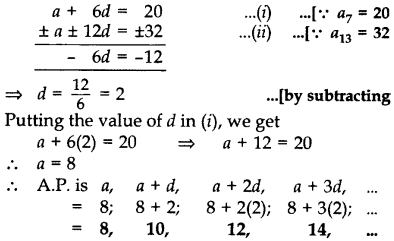Is the 10th term from end of the A.P. 4,9, 14, …, 254., 209?

જવાબ : Yes

Common difference d = 9 – 4
= 14 – 9 = 5
Given that the last term, l = 254, n = 10
nth term from the end = l – (n – 1) d
∴ 10th term from the end = 254 – (10 – 1) × 5
= 254 – 45 = 209

Find how many two-digit numbers are divisible by 6? (2011OD)

જવાબ : 6
Solution:
12, 18, 24, …,96
Here,First term a = 12, common diff. d = 18 – 12 = 6, an = 96
a + (n – 1)d = an
∴ 12 + (n – 1)6 = 96
⇒ (n − 1)6 = 96 – 12 = 84
⇒ n – 1 =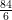= 14
⇒ n = 14 + 1 = 15
∴ There are 15 two-digit numbers divisible by 6.

How many natural numbers are there between 200 and 500, which are divisible by 7? (2011OD)

જવાબ : 43
Solution:
203, 210, 217, …, 497
Here, First term a = 203, common diff. d = 210 – 203 = 7, an = 497
∴ a + (n – 1) d = an
203 + (n – 1) 7 = 497
(n – 1) 7 = 497 – 203 = 294
n – 1 =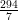= 42 ∴ n = 42 + 1 = 43
∴ There are 43 natural nos. between 200 and 500 which are divisible by 7.

How many two-digit numbers are divisible by 3? (2012OD)

જવાબ : 30
Solution:
Two-digit numbers divisible by 3 are:
12, 15, 18, …, 99
Here, First term a = 12, common diff. d = 15 – 12 = 3, an = 99
∴ a + (n – 1)d = an
12 + (n – 1) (3) = 99
(n – 1) (3) = 99 – 12 = 87
n – 1 =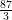= 29
∴ n = 29 + 1 = 30
∴ There are 30 such numbers.

Are there total 128 three-digit natural numbers are divisible by 7?

જવાબ : Yes
Solution:
“3 digits nos.” are 100, 101, 102, …, 999
3 digits nos. “divisible by 7” are:
105, 112, 119, 126, …, 994
first term a = 105, common diff. d = 7, an = 994, n = ?
As a + (n – 1)d = 994 = an
∴ 105 + (n – 1)7 = 994
(n − 1)7 = 994 – 105
(n – 1) =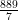= 127
∴ n = 127 + 1 = 128

Are there total 100 three-digit natural numbers which are divisible by 9?

જવાબ : Yes
Solution:
To find: Number of terms of A.P., i.e., n.
A.P. = 108 + 117 + 126 + … + 999
a = 108
d = 117 – 108 = 9
an = 999
a + (n – 1)d = an
∴ 108 + (n – 1) 9 = 999
⇒ (n − 1) 9 = 999 – 108 = 891
⇒ (n − 1) =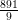= 99
∴ n = 99 + 1 = 100

Find the number of natural numbers between 101 and 999 which are divisible by both 2 and 5. (2014OD)

જવાબ : 89
Solution:
Numbers divisible by both 2 and 5 are 110, 120, 130, …, 990.
Here first term a = 110, common diff. d = 120 – 110 = 10, an = 990
As a + (n – 1)d = an = 990
110 + (n – 1)(10) = 990
(n – 1)(10) = 990 – 110 = 880
(n − 1) == 88
∴ n = 88 + 1 = 89

Do in an AP with first term a and common difference d, the nth term is given by an​=a+(n−1)d ?

જવાબ : Yes

State whether the statement True or False : 10th term of A.P. 10, 20, 30, 40 ......is 100

જવાબ : True

State whether the statement True or False : 20th term of A.P. whose first term = 10, common difference = 3 is 67

જવાબ : True

State whether the statement True or False : The number of bacteria in a certain food item after each second forms an AP. when they double in every second

જવાબ : False

State whether the statement True or False : The amount of money in the account of Varun at the end of every year forms an AP when Rs 1000 is deposited at simple interest of 10% per annum

જવાબ : True

If the nth term of the A. P. 9, 7, 5.... is same as the nth term of the A. P. 15, 12, 9...... find n

જવાબ : n=7

Which term of the A. P. 3, 15, 27, 39...... will be 132 more than its 54th term?

જવાબ : 65th

Which term of the arithmetic progression 5, 15, 25, ----- will be 130 more than its 31st term?

જવાબ : 44th

In an A. P, the sum of first ten terms is -150 and the sum of its next ten terms are -550. Find the A. P.

જવાબ : 6, 2, -2, -6 …….

In an A. P., the first term is 2, the last term is 29 and the sum of the terms is 155. Find the common difference of the A. P.

જવાબ : d=3

The first and the last term of an A. P. are 17 and 350 respectively. If the common difference is 9, how many terms are there and what is their sum?

જવાબ : n=38 & Sn =6973

In an A. P. the first term is 8, nth term is 33 and the sum to first n terms is 123. Find n and d, the common differences.

જવાબ : n=6 & d=5

In an A. P., the sum of first n terms is 3n2/2+13n/2. Find its 25th term.

જવાબ : T25=S25−S24T25=S25−S24 = [3(25)2 /2] +[13×25]/2−3(24)2/2 + [13×24]/2= 80

The 4th term of an A.P is equal to 3 times the first term and the 7th term exceeds twice the 3rd term by 1. Find the A.P.

જવાબ : The AP formed is 3, 5, 7, 9, .........

Find the value of the middle most term (s) of the AP
-11, -7, -3,..., 49

જવાબ : middle most terms will 8 and 9
T8=−11+(8−1)4=17T8=−11+(8−1)4= 17
T9=−11+(9−1)4=21T9=−11+(9−1)4= 21

Find the sum
x−yx+y+3x−2yx+y+5x−3yx+y+x−yx+y+3x−2yx+y+5x−3yx+y+..upto 11 terms

જવાબ : 11(11x−6y)/x+y

If the numbers n - 2, 4n - 1 and 5n + 2 are in AP, find the value of n.

જવાબ : 1

State whether the statement True or False : The fee charged every month by a school from Classes I to XII forms an AP, when the monthly fee for Class I is Rs 250, and it increases by Rs 50 for the next higher class.

જવાબ : True

State whether the statement True or False : The fee charged from a student every month by a school for the whole session forms an AP, when the monthly fee is Rs 400.

જવાબ : False

State whether the statement True or False : Is the sequence 5, 10, 15, 20,25...... an A.P.? If yes, find the Common Difference.

જવાબ : Yes, d=5

State whether the statement True or False : Is the sequence  2, 2, 2, 2,2...... an A.P.? If yes, find the Common Difference.

જવાબ : Yes, d=0

State whether the statement True or False : Is the sequence  2, 4, 8, 16,32...... an A.P.? If yes, find the Common Difference.

જવાબ : No

State whether the statement True or False : Is the sequence  3, 14, 25, 36......  an A.P.? If yes, find the Common Difference.

જવાબ : Yes, d=11

State whether the statement True or False : Is the sequence  11, 8, 5, 2, ..... an A.P.? If yes, find the Common Difference.

જવાબ : Yes, d=-3

Write next two terms of given A.P. -3, -5, -7,-9......

જવાબ : Next 2 terms are -11, -13

For what value of a, are (2a-1), 7 and 3a three consecutive terms of an A.P?

જવાબ : 3

How many multiples of 4 lie between 10 and 250?

જવાબ : 60

If Sn, the sum of first n terms of an A.P is given by
Sn=3n2−4nSn=3n2−4n
then find its nth term

જવાબ : 6n -7

Find the sum of the following A.P 1 + 3 + 5 + ..... + 199.

જવાબ : 10000

Solve the equation:
1+4+7+10+...+x=2871+4+7+10+...+x=287

જવાબ : 40

If pth, qth and rth terms of an A.P. are a, b, c respectively, then show that (a – b)r + (b – c)p+ (c – a)q = 0. (2011D)

જવાબ : Let A be the first term and D be the common difference of the given A.P.
pth term = A + (p – 1)D = a …(A)
qth term = A + (q – 1)D = b …(B)
rth term = A + (r – 1)D = c … (C)
L.H.S. = (a – b)r + (b – c)p + (c – a)q
= [A + (p – 1)D – (A + (q – 1)D)]r + [A + (q – 1)D – (A + (r – 1)D)]p + [A + (r – 1)D – (A + (p – 1)D)]q
= [(p – 1 – q + 1)D]r + [(q – 1 – r + 1)D]p + [(r – 1 – p + 1)D]q
= D[(p – q)r + (q – r)p + (r – p)q]
= D[pr – qr + qp – rp + rq – pq]
= D = 0 = R.H.S.

The 17th term of an AP is 5 more than twice its 8th term. If the 11th term of the AP is 43, then find its nth term. (2012D)

જવાબ :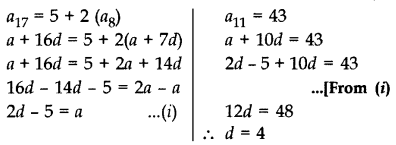From (i),
a = 2(4) – 5 = 8 – 5 = 3
As an = a + (n – 1) d
an = 3 + (n – 1) 4 = 3 + 4n – 4
an = (4n – 1)

The 15th term of an AP is 3 more than twice its 7th term. If the 10th term of the AP is 41, then find its nth term. (2012D)

જવાબ :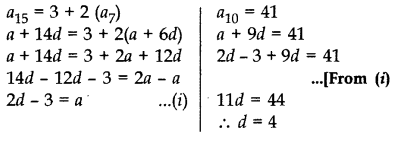From (i),
a = 2(4) – 3
= 8 – 3 = 5
nth term = a + (n – 1) d

nth term = 5 + (n – 1) 4
= 5 + 4n – 4 = (4n + 1)

The 16th term of an AP is 1 more than twice its 8th term. If the 12th term of the AP is 47, then find its nth term. (2012D)

જવાબ :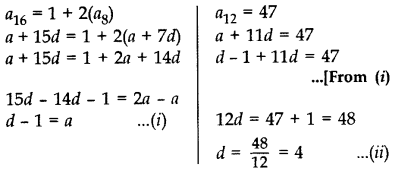From (A) and (B), a = 4 – 1 = 3
As nth term = a + (n – 1) d
nth term = 3 + (n – 1) 4
= 3 + 4n -4 = 4n -1

Find the 60th term of the AP 8, 10, 12, …, if it has a total of 60 terms and hence find the sum of its last 10 terms. (2015OD)

જવાબ : a = 8, d = a2 – a1 = 10 – 8 = 2, n = 60
a60 = a + 59d = 8 + 59(2) = 126
Sum of its last 10 terms = S60 – S50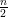(a + an) –(2a + (n – 1)d)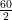(8 + a60) –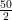(2 × 8 + (50 – 1)2)
= 30 (8 + 126) – 25 (16 + 98)
= 4020 – 25 × 114
= 4020 – 2850 = 1170

An Arithmetic Progression 5, 12, 19, … has 50 terms. Find its last term. Hence find the sum of its last 15 terms. (2015OD)

જવાબ : Let, ‘a’ be the first term and ‘d’ be the common difference of A.P. respectively,
a = 5, d = 12 – 5 = 7, n = 50
an = a + (n – 1)d
a50 = 5 + 49(7) = 5 + 343 = 348
Sum of its last 15 terms = S50 – S35(a + an) –(2a + (n − 1)d)(5 + 348) –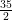[2(5) + (35 – 1)7]
= 25(353) –(10 + 238)
= 8825 – 35 × 124
= 8825 – 4340 = 4485

If the sum of first 4 terms of an A.P. is 40 and that of first 14 terms is 280, find the sum of its first n terms. (2011D)

જવાબ : Sn =[2a + (n − 1) d] …(A)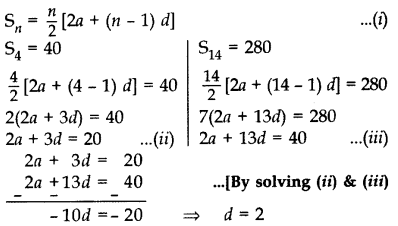Putting the value of d = 2 in (B), we get a = 7
Sn =[2(7) + (n – 1). 2].2 [7 + n – 1]
= n (n + 6) or n2 + 6n

A sum of ₹1,600 is to be used to give ten cash prizes to students of a school for their overall academic performance. If each prize is ₹20 less than its preceding prize, find the value of each of the prizes. (2012OD)

જવાબ : Here, Sum of 10 prizes = 1600

S10 = 1600, d = -20, n = 10
Sn =(2a + (n – 1)d]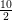[2a + (10 – 1)(-20)] = 1600
2a – 180 = 320
2a = 320 + 180 = 500
a = 250
Hence, 1st prize = a = ₹250
2nd prize = a2 = a + d = 250 + (-20) = ₹230
3rd prize = a3 = a2 + d = 230 – 20 = ₹210
4th prize = a4 = a3 + d = 210 – 20 = ₹190
5th prize = a5 = a4 + d = 190 – 20 = ₹170
6th prize = a6 = a5 + d = 170 – 20 = ₹150
7th prize = a7 = a6 + d = 150 – 20 = ₹130
8th prize = a8 = a7 + d = 130 – 20 = ₹110
9th prize = a9 = a8 + d = 110 – 20 = ₹590
10th prize = a10 = a9 + d = 90 – 20 = ₹70
= ₹ 1,600

The first and the last terms of an A.P. are 8 and 350 respectively. If its common difference is 9, how many terms are there and what is their sum? (2011D)

જવાબ : Here a = 8, l = 350, d = 9
As we know, a + (n − 1) d = a2
8 + (n – 1) 9 = 350
(n − 1) 9 = 350 – 8 = 342
n – 1 =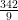= 38
n = 38 + 1 = 39
There are 39 terms.
Sn =(a + l)
S39 =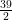(8 + 350) =× 358
= 39 × 179 = 6981

Sum of the first 20 terms of an AP is -240, and its first term is 7. Find its 24th term. (2012D)

જવાબ : Given: a = 7, S20 = -240
Here, Sn =[2a + (n – 1)d]
S20 =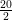[2(7) + (20 – 1)d]
-240 = 10(14 + 19d)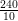= 14 + 19d = -24
19d = -24 – 14 = -38
d =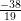= -2 …(A)
Again, an = a + (n – 1)d
a24 = 7 + (24 – 1) (-2) … [From (A)
= 7 – 46 = -39

Find the common difference of an A.P. whose first term is 5 and the sum of its first four terms is half the sum of the next four terms. (2012OD)

જવાબ : Here a = 5 … (A)
Here, a1 + a2 + a3 + a4 =(a5 + a6 + a7 + a8)
a + (a + d) + (a + 2d) + (a + 3d)[(a + 4d) + (a + 5d) + (a + 6d) + (a + 780] …[an = a(n – 1)d
4a + 6d =(4a + 22d)
8a + 12d = 4a + 22d
8a – 4a = 22d – 12d
4a = 100 4(5) = 10d
d =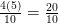= 2 …[From (A)
Common difference, d = 2

If the sum of the first 7 terms of an A.P. is 119 and that of the first 17 terms is 714, find the sum of its first n terms. (2012OD)

જવાબ : As Sn =[2a + (n − 1)d]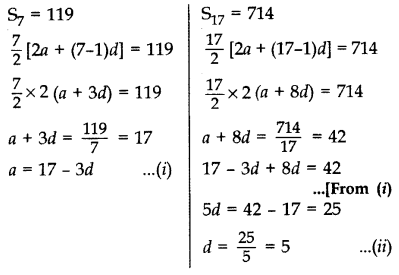S2 = 119
From (A) and (B), a = 17 – 3(5) = 17 – 15 = 2
Sn =[2(2) + (n – 1)5][4 + 5n – 5](5n – 1)

The 24th term of an AP is twice its 10th term. Show that its 72nd term is 4 times its 15th term. (2013D)

જવાબ : Solution:
a24 = 2 (10) … [Given
a + 23d = 2 (a +9d) [ an = a + (n – 1)d)
23d = 2a + 18d – a
23d – 18d = a a = 5d …(A)
To prove: a72 = 4 (a15)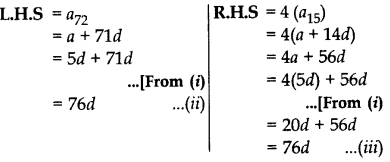From (ii) and (iii), L.H.S = R.H.S …Hence Proved

Find the number of terms of the A.P. -12, -9, – 6, …, 21. If 1 is added to each term of this A.P., then find the sum of all terms of the A.P. thus obtained. (2013D)

જવાબ : Given that, a = -12, d=-9 + 12 = 3
Here, an = a + (n – 1)d = 21
-12 + (n – 1).3 = 21
(n – 1).3 = 21 + 12 = 33
n – 1 = 11
Total number of terms,
n = 11 + 1 = 12
New A.P. is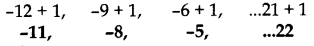Here 1st term, a = -11
Common difference, d = -8 + 11 = 3;
Last term, an = 22; Number of terms, n= 12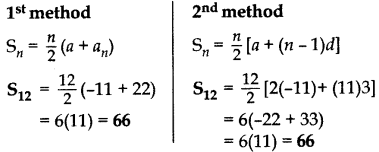In an AP of 50 terms, the sum of first 10 terms is 210 and the sum of its last 15 terms is 2565. Find the AP. (2014D)

જવાબ : Here, n = 50,
Here, S10 = 210(2a + 9d) = 210 ….[Sn =[2a+(n – 1)2]
5(2a + 9d) = 210
2a + 9d =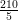= 42
2a = 42 – 9d a =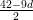…(A)
Now, 50 = (1 + 2 + 3 + …) + (36 + 37 + … + 50) Sum = 2565
Sum of its last 15 terms = 2565 …[Given
S50 – S35 = 2565(2a + 49d) –(2a + 34d) = 2565
100a + 2450d – 70a – 1190d = 2565 × 2
30a + 1260d = 5130
3a + 1260 = 513 …[Dividing both sides by 10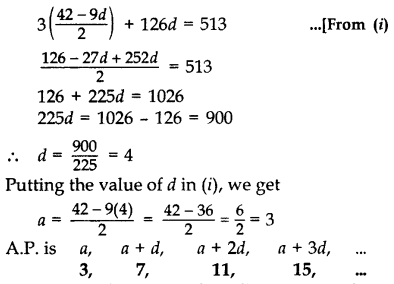If the ratio of the sum of the first n terms of two A.Ps is (7n + 1): (4n + 27), then find the ratio of their 9th terms. (2017OD)

જવાબ :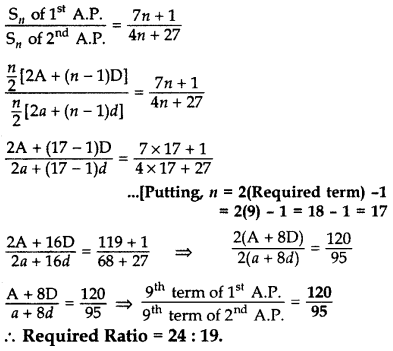In a school, students decided to plant trees in and around the school to reduce air pollution. It was decided that the number of trees, that each section of each class will plant, will be double of the class in which they are studying. If there are 1 to 12 classes in the school and each class has two Sections, find how many trees were planted by the students. (2014OD)

જવાબ : Classes: 1 + I + II + … + XII
Sections: 2(I) + 2(II) + 2(III) + … + 2(XII)
Total no. of trees
= 2 + 4 + 6 … + 24
= (2 × 2) + (2 × 4) + (2 × 6) + … + (2 × 24)
= 4 + 8 + 12 + … + 48
:: S12 =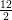(4 + 48) = 6(52) = 312 trees

Ramkali required ₹500 after 12 weeks to send her daughter to school. She saved ₹100 in the first week and increased her weekly saving by ₹20 every week. Find whether she will be able to send her daughter to school after 12 weeks. (2015D)

જવાબ : Money required by Ramkali for admission of her daughter = ₹2500
A.P. formed by saving
100, 120, 140, … upto 12 terms ….(A)
Let, a, d and n be the first term, common difference and number of terms respectively. Here, a = 100, d = 20, n = 12
Sn =(2a + (n − 1)d)
S12 =(2(100) + (12 – 1)20)
S12 =[2(100) + 11(20)] = 6 = ₹2520

The sum of first n terms of an A.P. is 5n2 + 3n. If its mth term is 168, find the value of m. Also find the 20th term of this A.P. (2013D)

જવાબ :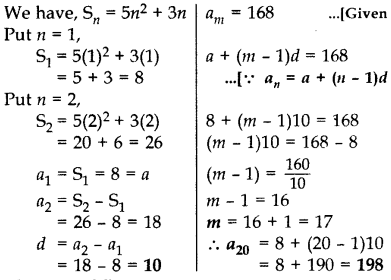The sum of first m terms of an AP is 4m2 – m. If its nth term is 107, find the value of n. Also find the 21st term of this A.P. (2013D)

જવાબ : We have, Sm = 4m2 – m
Put m = 1,
S1 = 4(1)2 – (1)
= 4 – 1 = 3
Put m = 2,
S2 = 4(2)2 – 2
= 16 – 2 = 14
a1 = S1 = 3 = a
a2 = S2 – S1 = 14 – 3 = 11
d = a2 – a1
d = 11 – 3 = 8
an = a + (n – 1)d = 107 …[Given in the ques.
3+ (n – 1)8 = 107
(n – 1)8 = 107 – 3 = 104
(n – 1) =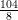= 13
n = 13 + 1 = 14
a21 = a + 20d
= 3 + (20)8
= 3 + 160 = 163

The sum of first g terms of an A.P. is 63q – 3q2. If its pth term is -60, find the value of p. Also find the 11th term of this A.P. (2013D)

જવાબ : We have, Sq = 63q – 3q2
Put q = 1 in the equation
S7 = 63(1) – 3(1)2
= 63 – 3 = 60
Put q = 2 in the equation
S2 = 63(2) – 3(2)2
= 126 – 12 = 114
a = a1 = S1 = 60
a2 = S2 – S71 = 114 – 60 = 54
d = a2 – a1 = 54 – 60 = -6
pth term = -60
a + (p – 1)d = -60
60 + (p – 1)(-6) = -60
(p – 1)(-6) = -60 – 60 = -120
(p – 1) =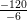= 20
p= 20 + 1 = 21
a11 = a + 10d
= 60 + 10(-6)
= 60 – 60 = 0

### There are No Content Availble For this Chapter

Find the 10th term

 1 1,3,5,7…. A 57 2 2,4,6,8…. B 51 3 2,7,12,17…. C 19 4 21,24,27,30…. D 20

જવાબ :

1-C, 2-D, 3-A, 4-B

Find the nth term

 1 2,6,10,14…. A 8+10n 2 13,9,5,1…. B 4n-2 3 18,28,38,48…. C 26-n 4 25,24,23,22,…. D 17-4n

જવાબ :

1-B, 2-D, 3-A, 4-C

Find the 2nd term from last

 1 -2,5,…….,68 A 900 2 -100,0,….., 1000 B 98 3 28,30,…… , 100 C 15 4 75,45,….-15 D 61

જવાબ :

1-D, 2-A, 3-B, 4-C

 1 43,__, 51,55,59 A 81 2 75,78, __, 94 B 47 3 __, 27,30,33 C -164 4 82, 0, -82, __ D 24

જવાબ :

1-B, 2-A, 3-D, 4-C

Find the common differences

 1 89,85,81,77 A 11 2 100, 89, 78, 67 B -4 3 100, 111,122,133 C 4 4 89, 93, 97, 101 D -11

જવાબ :

1-B, 2-D, 3-A, 4-C

Find a4

 1 8+24n A 7 2 23-4n B 104 3 4+7n C -8 4 32-10n D 32

જવાબ :

1-B, 2-A, 3-D, 4-C

Find the 9th term from last

 1 75+20n A 5 2 32-3n B 54 3 4n-90 C 80 4 20n-100 D 255

જવાબ :

1-D, 2-A, 3-B, 4-C

Find the nth term

 1 a=2, d=4 A 12-2n 2 a=4, d=2 B 14-4n 3 a=10, d=-2 C 4n-2 4 a=10, d=-4 D 2+2n

જવાબ :

1-C, 2-D, 3-A, 4-B

Find the S8

 1 1,3,5,7…. A 252 2 4,6,8,10…. B 156 3 2,7,12,17…. C 88 4 21,24,27,30…. D 72

જવાબ :

1-D, 2-C, 3-B, 4-A

Find S4

 1 8+24n A 68 2 23-4n B 224 3 4+7n C 64 4 31-10n D 72

જવાબ :

1-B, 2-A, 3-D, 4-C

### Take a Test

Choose your Test :

### Arithmetic Progressions

Math
Chapter 05 : Arithmetic Progressions

### Browse & Download CBSE Books For Class 10 All Subjects

The GSEB Books for class 10 are designed as per the syllabus followed Gujarat Secondary and Higher Secondary Education Board provides key detailed, and a through solutions to all the questions relating to the GSEB textbooks.

The purpose is to provide help to the students with their homework, preparing for the examinations and personal learning. These books are very helpful for the preparation of examination.

For more details about the GSEB books for Class 10, you can access the PDF which is as in the above given links for the same.